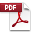# MATH BRAINTEASERS

Reproducible activity book

\$16.95

Order Code: GDY511    ISBN : 9781596470361
Connect with a representative to create a custom curriculum for your district.

By April Blakely. A coin is tossed three times. What is the probability of flipping heads, heads, tails? A dog is tied to the corner of a house by a leash 30 feet long. Over how much area can the dog run? More than 100 problem-solving exercises challenge kids to figure out mathematical problems dealing with probability, geometry, percents, number theory, fractions, algebraic expressions, and other math concepts. Each problem is stated on a reproducible handout. Grades 6–12. Answer key. Illustrated. 8½" x 11". Good Year. 90 pages. ©1997.Sample pages